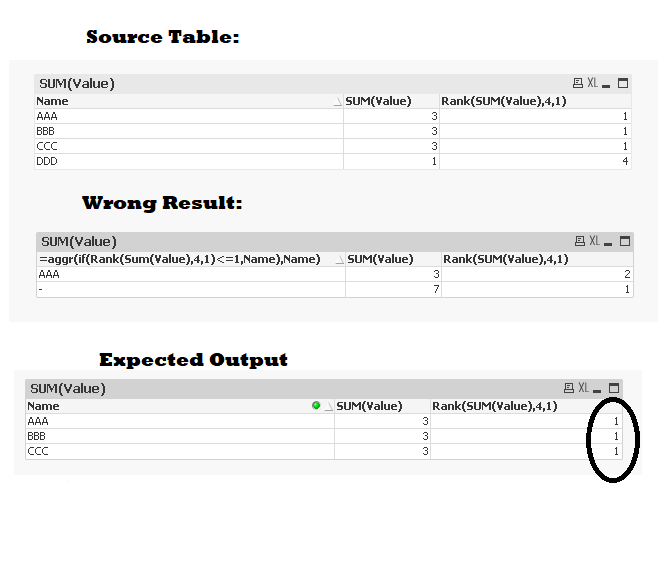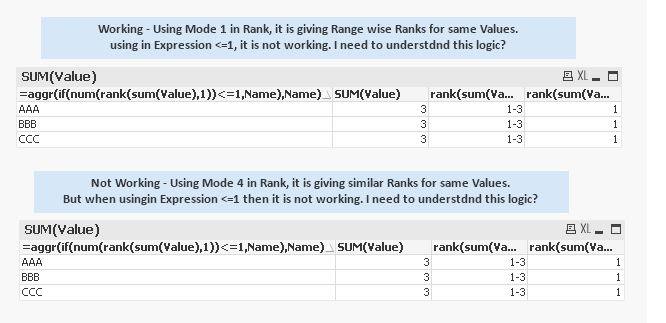# QlikView Scripting

Discussion Board for collaboration on QlikView Scripting.

Announcements
Modernize Your QlikView Deployment webinar, Nov. 3rd. REGISTER
cancel
Showing results for
Did you mean:
HighlightedContributor III

## Rank Logic & Dimension Limits both are giving wrong result for similar Ranks

Hi All,

First, I tried with Dimension Limits but it wqas giving wrong result for similar Ranks. I want to see all Rank1 Names but it was giving only 1.

Then I tried Rank Function.

I need all the Rank 1 Names in my Chart but using below expression

in calculated dimension is giving me only 1 result which is wrong.

=aggr(if(Rank(Sum(Value),4,1)<=1,Name),Name)

Here is the sample snapshot:'Need help in understanding this Rank & fixing this issue.

Regards,

Eric

Labels (3)

• ### Rank

1 Solution

Accepted Solutions
HighlightedMVP

@erric3210  try below

``=num(rank(total sum(Sales),1))``
7 Replies
HighlightedMVP

@erric3210  try below

``=num(rank(total sum(Sales),1))``
HighlightedContributor III

Hi Kush,

Thanks for the solution. It worked.

Can you please explain me what is the role of TOTAL here.

I've tried with your expression, without Total also it is working

and if possible can you please make me understand that why below expression was not working

=aggr(if(Rank(Sum(Value),4,1)<=1,Name),Name)

Regards,

Eric

HighlightedContributor III

Hi Kush,

Can you please make me understand why Mode 4 is not working and 1 is working. I can clearly see that Mode 4 is giving similar values for Same value and it's not using range but still it's not going right result when using <=1 condition and mode 1 is working when it has values in ranges. how come it is showing values 5 values in <=1 when in actual it is representing them as 1-5.

Is 1-5 internally interpreted as 1 and Mode1 rank 1 are interpreted differently internally?

Here is the snapshot:Regards,

Eric

HighlightedMVP

You can ignore total keyword and you can also use below where mode =1 provides lowest rank on all rows and format=1 gives low value on all rows

``````=rank(total sum(Sales),1,1))

or

=rank( sum(Sales),1,1))``````

HighlightedContributor III

Hi Kush,

Thank you for the solution & explanation.

Kush, earlier you've used only 1 value in the end  (=num(rank(total sum(Sales),1)))

and also removed Num function from 2nd expression.

but now you're using 2 values in the end (=rank(total sum(Sales),1,1)))

What is the difference b/w them.

Regards,

Eric

HighlightedMVP

@erric3210  only difference is in second solution I have used second format parameter which you can use to avoid using num function in first solution

HighlightedContributor III

Great....!! Thanks Kush..........!!